# RD Sharma Solutions for Class 11 Chapter 19 - Arithmetic Progressions Exercise 19.7

In this section, we shall discuss some problems based upon the applications of arithmetic progressions. We all know that board exams are very important in a student’s life. To achieve their desired goals, students require a lot of practice in order to solve problems more confidently in the exam. To make this possible, subject experts have formulated the solutions, as per the requirements of students. RD Sharma Class 11 Maths Solutions pdf is provided here. Students can easily download the pdf and start practising offline to come out with flying colours.

## Download the pdf of RD Sharma Solutions for Class 11 Maths Exercise 19.7 Chapter 19 – Arithmetic Progressions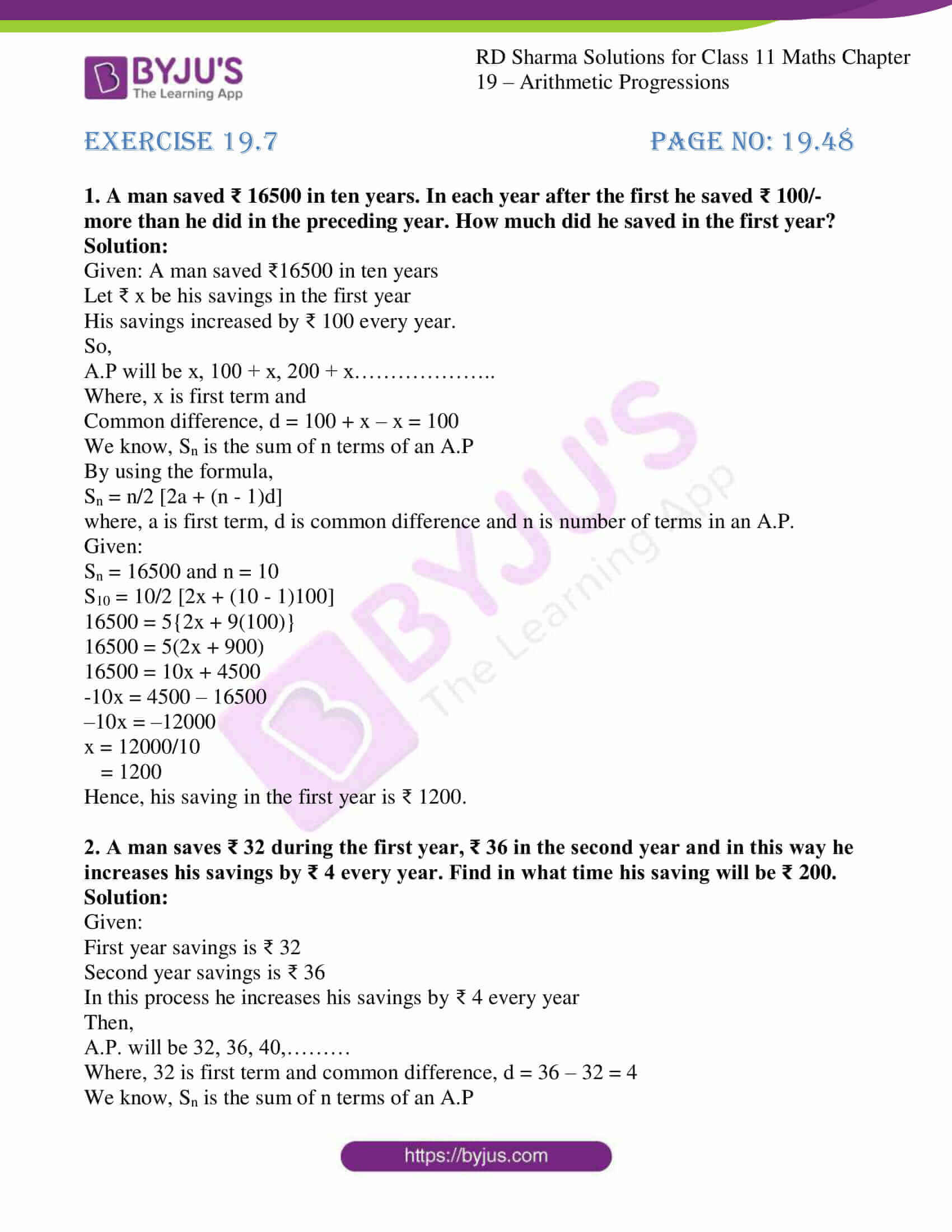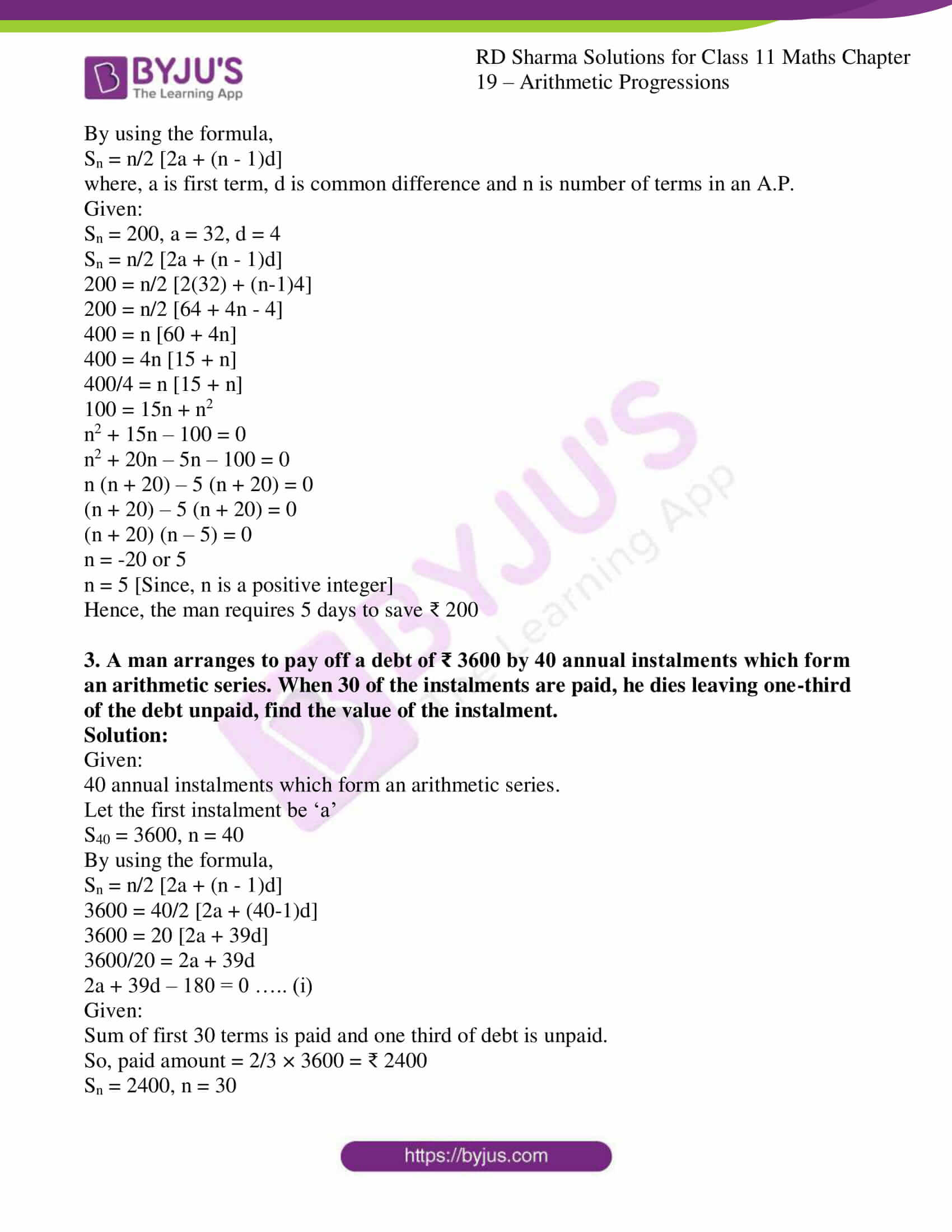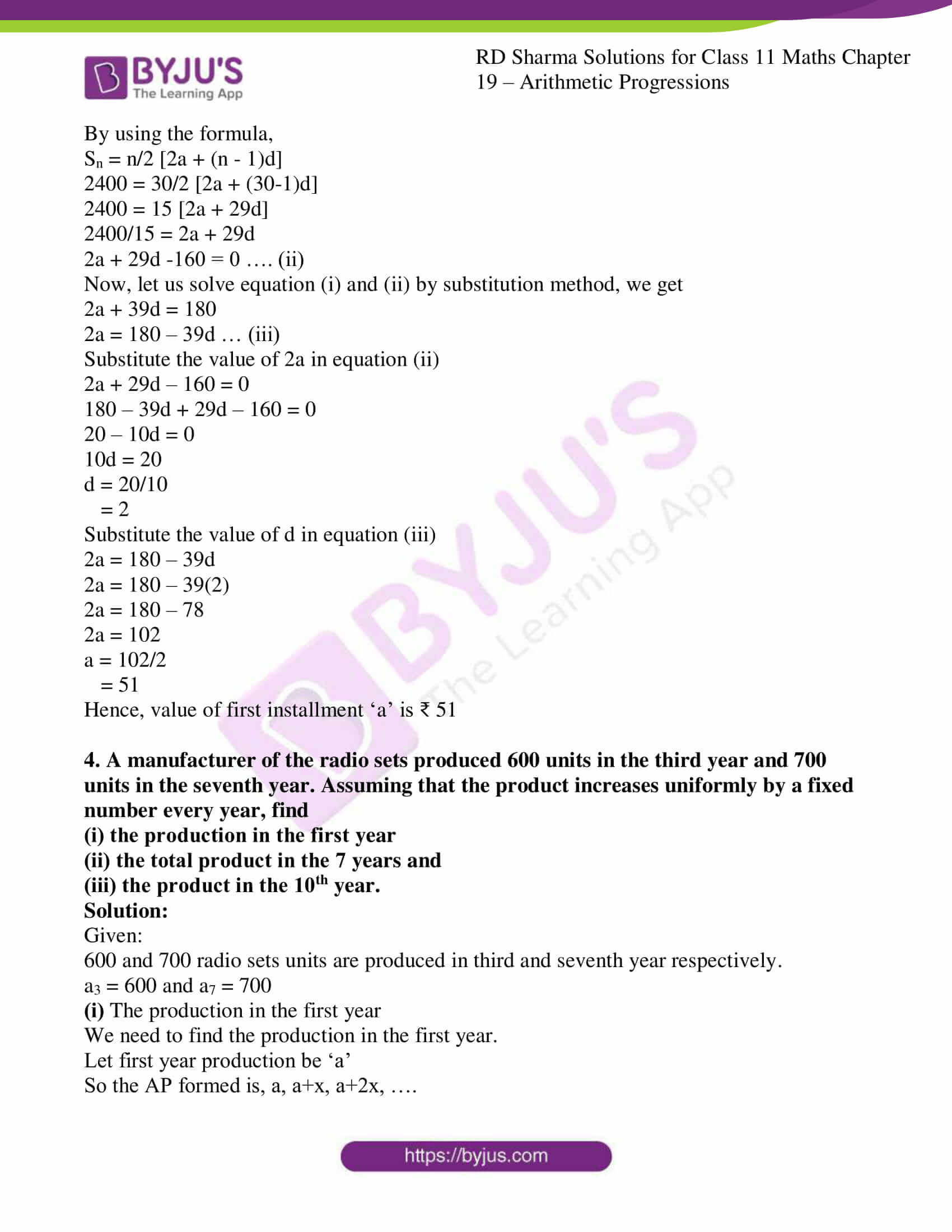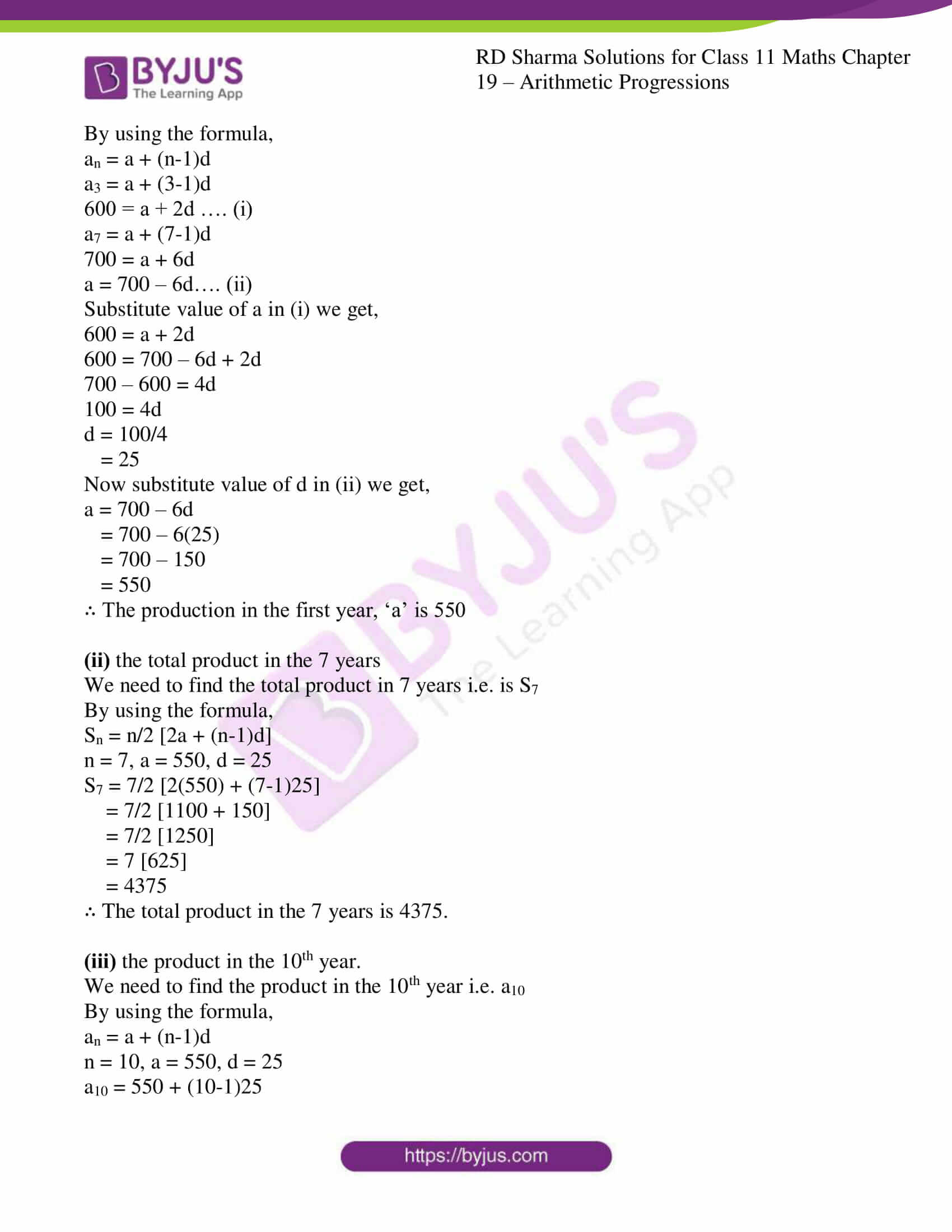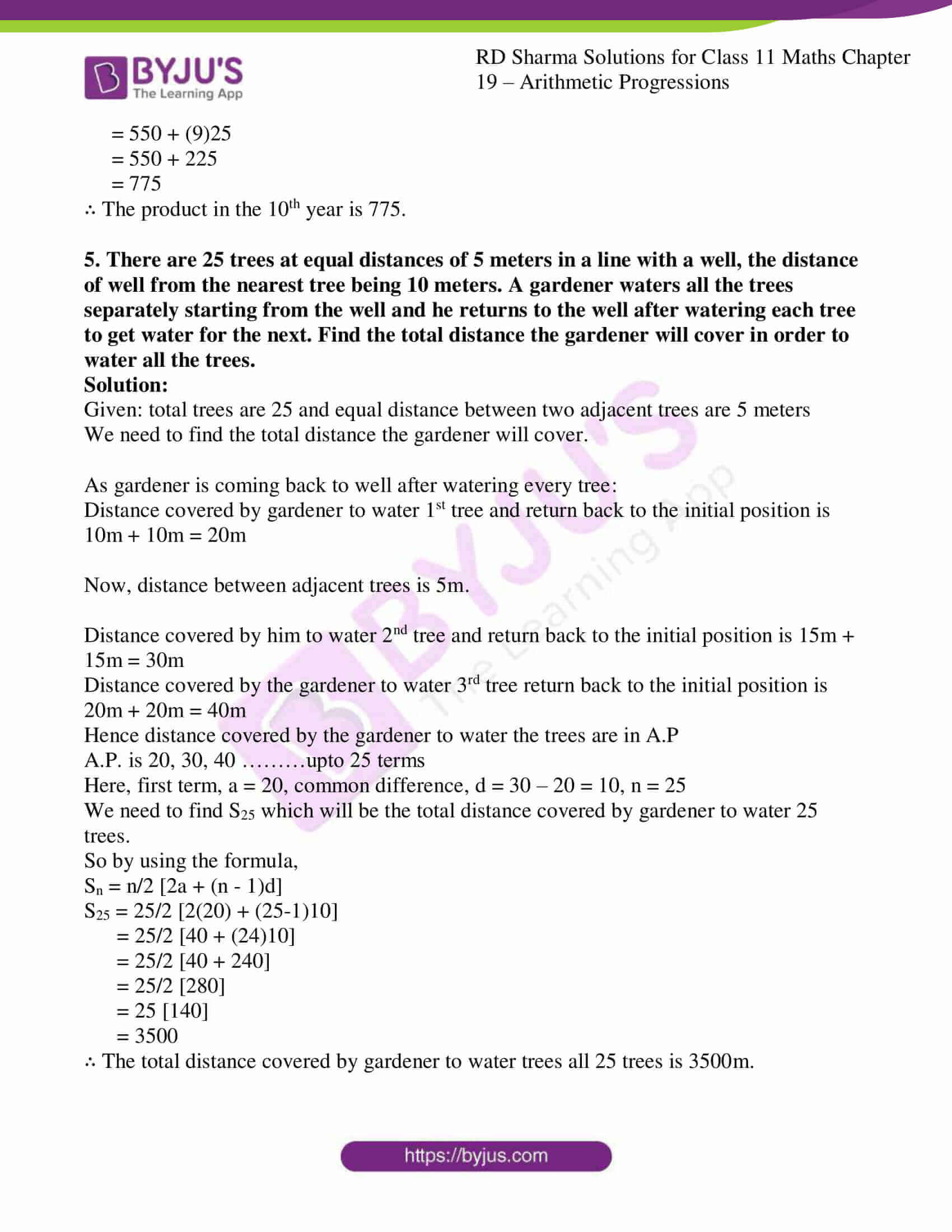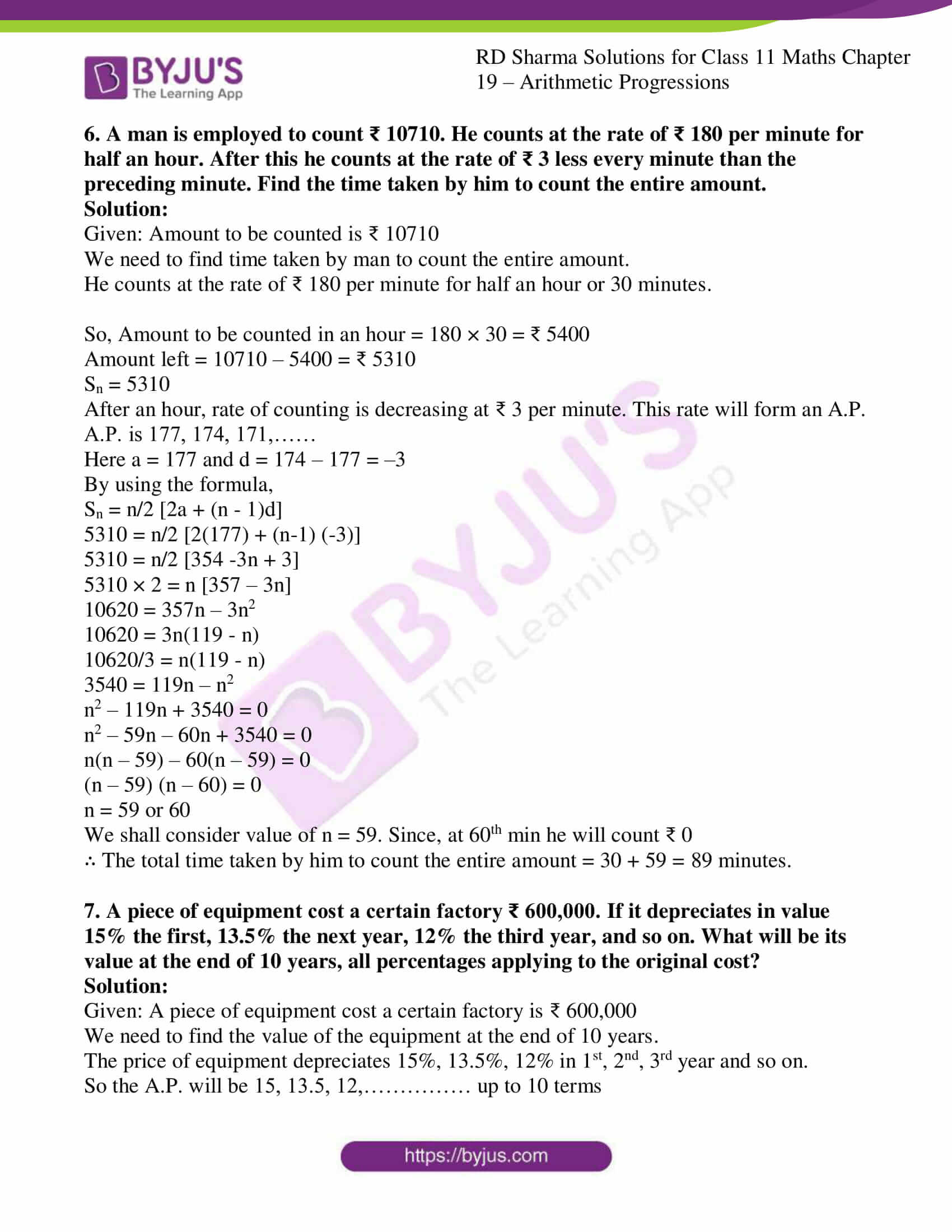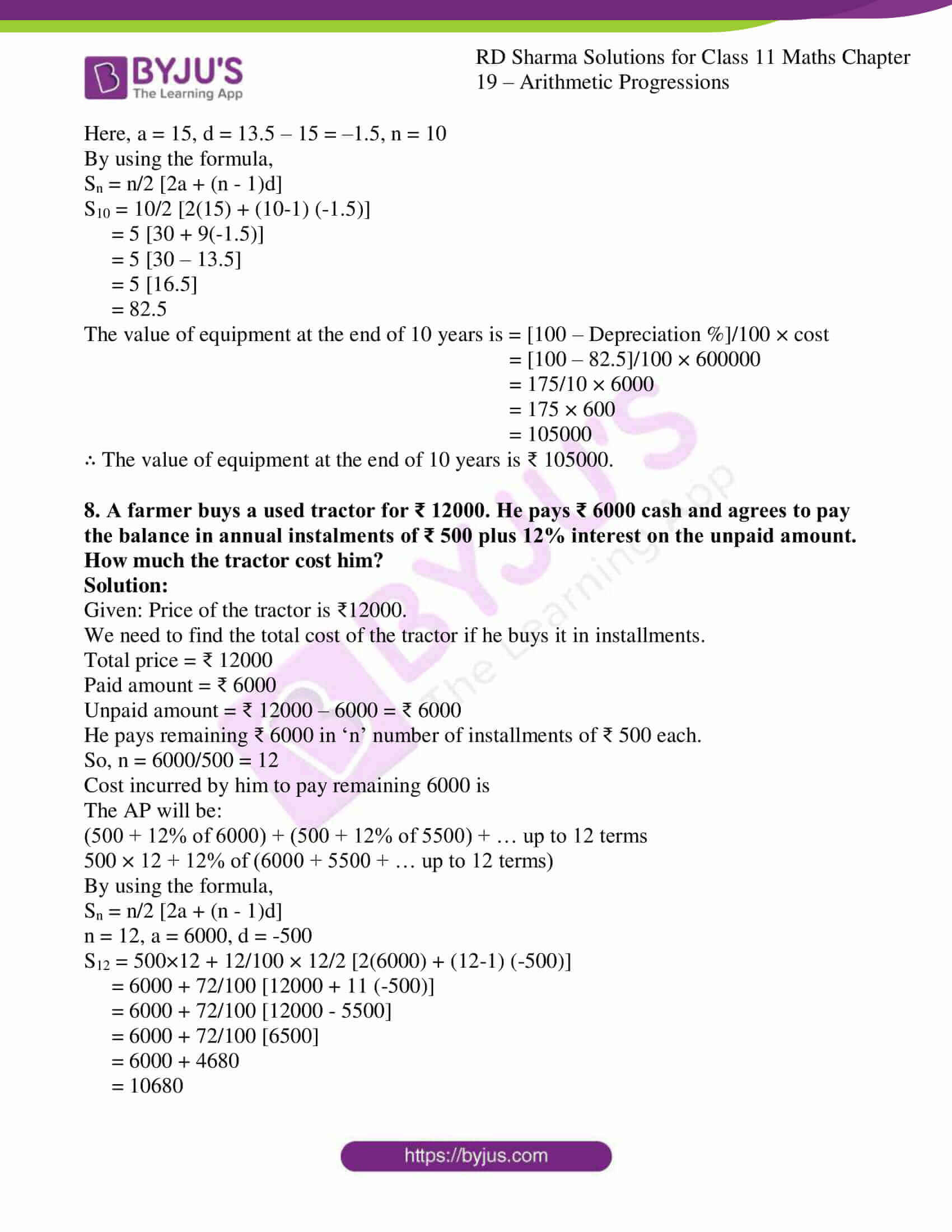### Access answers to RD Sharma Solutions for Class 11 Maths Exercise 19.7 Chapter 19 – Arithmetic Progressions

1. A man saved ₹ 16500 in ten years. In each year after the first he saved ₹ 100/- more than he did in the preceding year. How much did he saved in the first year?

Solution:

Given: A man saved ₹16500 in ten years

Let ₹ x be his savings in the first year

His savings increased by ₹ 100 every year.

So,

A.P will be x, 100 + x, 200 + x………………..

Where, x is first term and

Common difference, d = 100 + x – x = 100

We know, Sn is the sum of n terms of an A.P

By using the formula,

Sn = n/2 [2a + (n – 1)d]
where, a is first term, d is common difference and n is number of terms in an A.P.

Given:

Sn = 16500 and n = 10

S10 = 10/2 [2x + (10 – 1)100]
16500 = 5{2x + 9(100)}

16500 = 5(2x + 900)

16500 = 10x + 4500

-10x = 4500 – 16500

–10x = –12000

x = 12000/10

= 1200

Hence, his saving in the first year is ₹ 1200.

2. A man saves ₹ 32 during the first year, ₹ 36 in the second year and in this way he increases his savings by ₹ 4 every year. Find in what time his saving will be ₹ 200.

Solution:

Given:

First year savings is ₹ 32

Second year savings is ₹ 36

In this process he increases his savings by ₹ 4 every year

Then,

A.P. will be 32, 36, 40,………

Where, 32 is first term and common difference, d = 36 – 32 = 4

We know, Sn is the sum of n terms of an A.P

By using the formula,

Sn = n/2 [2a + (n – 1)d]
where, a is first term, d is common difference and n is number of terms in an A.P.

Given:

Sn = 200, a = 32, d = 4

Sn = n/2 [2a + (n – 1)d]

200 = n/2 [2(32) + (n-1)4]
200 = n/2 [64 + 4n – 4]

400 = n [60 + 4n]

400 = 4n [15 + n]

400/4 = n [15 + n]

100 = 15n + n2

n2 + 15n – 100 = 0

n2 + 20n – 5n – 100 = 0

n (n + 20) – 5 (n + 20) = 0

(n + 20) – 5 (n + 20) = 0

(n + 20) (n – 5) = 0

n = -20 or 5

n = 5 [Since, n is a positive integer]

Hence, the man requires 5 days to save ₹ 200

3. A man arranges to pay off a debt of ₹ 3600 by 40 annual instalments which form an arithmetic series. When 30 of the instalments are paid, he dies leaving one-third of the debt unpaid, find the value of the instalment.

Solution:

Given:

40 annual instalments which form an arithmetic series.

Let the first instalment be ‘a’

S40 = 3600, n = 40

By using the formula,

Sn = n/2 [2a + (n – 1)d]

3600 = 40/2 [2a + (40-1)d]

3600 = 20 [2a + 39d]

3600/20 = 2a + 39d

2a + 39d – 180 = 0 ….. (i)

Given:

Sum of first 30 terms is paid and one third of debt is unpaid.

So, paid amount = 2/3 × 3600 = ₹ 2400

Sn = 2400, n = 30

By using the formula,

Sn = n/2 [2a + (n – 1)d]

2400 = 30/2 [2a + (30-1)d]

2400 = 15 [2a + 29d]

2400/15 = 2a + 29d

2a + 29d -160 = 0 …. (ii)

Now, let us solve equation (i) and (ii) by substitution method, we get

2a + 39d = 180

2a = 180 – 39d … (iii)

Substitute the value of 2a in equation (ii)

2a + 29d – 160 = 0

180 – 39d + 29d – 160 = 0

20 – 10d = 0

10d = 20

d = 20/10

= 2

Substitute the value of d in equation (iii)

2a = 180 – 39d

2a = 180 – 39(2)

2a = 180 – 78

2a = 102

a = 102/2

= 51

Hence, value of first installment ‘a’ is ₹ 51

4. A manufacturer of the radio sets produced 600 units in the third year and 700 units in the seventh year. Assuming that the product increases uniformly by a fixed number every year, find
(і) the production in the first year
(іі) the total product in the 7 years and
(ііі) the product in the 10th year.

Solution:

Given:

600 and 700 radio sets units are produced in third and seventh year respectively.

a3 = 600 and a7 = 700

(і) The production in the first year

We need to find the production in the first year.

Let first year production be ‘a’

So the AP formed is, a, a+x, a+2x, ….

By using the formula,

an = a + (n-1)d

a3 = a + (3-1)d

600 = a + 2d …. (i)

a7 = a + (7-1)d

700 = a + 6d

a = 700 – 6d…. (ii)

Substitute value of a in (i) we get,

600 = a + 2d

600 = 700 – 6d + 2d

700 – 600 = 4d

100 = 4d

d = 100/4

= 25

Now substitute value of d in (ii) we get,

a = 700 – 6d

= 700 – 6(25)

= 700 – 150

= 550

∴ The production in the first year, ‘a’ is 550

(іі) the total product in the 7 years

We need to find the total product in 7 years i.e. is S7

By using the formula,

Sn = n/2 [2a + (n-1)d]

n = 7, a = 550, d = 25

S7 = 7/2 [2(550) + (7-1)25]

= 7/2 [1100 + 150]

= 7/2 

= 7 

= 4375

∴ The total product in the 7 years is 4375.

(ііі) the product in the 10th year.

We need to find the product in the 10th year i.e. a10

By using the formula,

an = a + (n-1)d

n = 10, a = 550, d = 25

a10 = 550 + (10-1)25

= 550 + (9)25

= 550 + 225

= 775

∴ The product in the 10th year is 775.

5. There are 25 trees at equal distances of 5 meters in a line with a well, the distance of well from the nearest tree being 10 meters. A gardener waters all the trees separately starting from the well and he returns to the well after watering each tree to get water for the next. Find the total distance the gardener will cover in order to water all the trees.

Solution:

Given: total trees are 25 and equal distance between two adjacent trees are 5 meters

We need to find the total distance the gardener will cover.

As gardener is coming back to well after watering every tree:

Distance covered by gardener to water 1st tree and return back to the initial position is 10m + 10m = 20m

Now, distance between adjacent trees is 5m.

Distance covered by him to water 2nd tree and return back to the initial position is 15m + 15m = 30m

Distance covered by the gardener to water 3rd tree return back to the initial position is 20m + 20m = 40m

Hence distance covered by the gardener to water the trees are in A.P

A.P. is 20, 30, 40 ………upto 25 terms

Here, first term, a = 20, common difference, d = 30 – 20 = 10, n = 25

We need to find S25 which will be the total distance covered by gardener to water 25 trees.

So by using the formula,

Sn = n/2 [2a + (n – 1)d]

S25 = 25/2 [2(20) + (25-1)10]

= 25/2 [40 + (24)10]

= 25/2 [40 + 240]

= 25/2 

= 25 

= 3500

∴ The total distance covered by gardener to water trees all 25 trees is 3500m.

6. A man is employed to count ₹ 10710. He counts at the rate of ₹ 180 per minute for half an hour. After this he counts at the rate of ₹ 3 less every minute than the preceding minute. Find the time taken by him to count the entire amount.

Solution:

Given: Amount to be counted is ₹ 10710

We need to find time taken by man to count the entire amount.

He counts at the rate of ₹ 180 per minute for half an hour or 30 minutes.

So, Amount to be counted in an hour = 180 × 30 = ₹ 5400

Amount left = 10710 – 5400 = ₹ 5310

Sn = 5310

After an hour, rate of counting is decreasing at ₹ 3 per minute. This rate will form an A.P.

A.P. is 177, 174, 171,……

Here a = 177 and d = 174 – 177 = –3

By using the formula,

Sn = n/2 [2a + (n – 1)d]

5310 = n/2 [2(177) + (n-1) (-3)]

5310 = n/2 [354 -3n + 3]

5310 × 2 = n [357 – 3n]

10620 = 357n – 3n2

10620 = 3n(119 – n)

10620/3 = n(119 – n)

3540 = 119n – n2

n2 – 119n + 3540 = 0

n2 – 59n – 60n + 3540 = 0

n(n – 59) – 60(n – 59) = 0

(n – 59) (n – 60) = 0

n = 59 or 60

We shall consider value of n = 59. Since, at 60th min he will count ₹ 0

∴ The total time taken by him to count the entire amount = 30 + 59 = 89 minutes.

7. A piece of equipment cost a certain factory ₹ 600,000. If it depreciates in value 15% the first, 13.5% the next year, 12% the third year, and so on. What will be its value at the end of 10 years, all percentages applying to the original cost?

Solution:

Given: A piece of equipment cost a certain factory is ₹ 600,000

We need to find the value of the equipment at the end of 10 years.

The price of equipment depreciates 15%, 13.5%, 12% in 1st, 2nd, 3rd year and so on.

So the A.P. will be 15, 13.5, 12,…………… up to 10 terms

Here, a = 15, d = 13.5 – 15 = –1.5, n = 10

By using the formula,

Sn = n/2 [2a + (n – 1)d]

S10 = 10/2 [2(15) + (10-1) (-1.5)]

= 5 [30 + 9(-1.5)]

= 5 [30 – 13.5]

= 5 [16.5]

= 82.5

The value of equipment at the end of 10 years is = [100 – Depreciation %]/100 × cost

= [100 – 82.5]/100 × 600000

= 175/10 × 6000

= 175 × 600

= 105000

∴ The value of equipment at the end of 10 years is ₹ 105000.

8. A farmer buys a used tractor for ₹ 12000. He pays ₹ 6000 cash and agrees to pay the balance in annual instalments of ₹ 500 plus 12% interest on the unpaid amount. How much the tractor cost him?

Solution:

Given: Price of the tractor is ₹12000.

We need to find the total cost of the tractor if he buys it in installments.

Total price = ₹ 12000

Paid amount = ₹ 6000

Unpaid amount = ₹ 12000 – 6000 = ₹ 6000

He pays remaining ₹ 6000 in ‘n’ number of installments of ₹ 500 each.

So, n = 6000/500 = 12

Cost incurred by him to pay remaining 6000 is

The AP will be:

(500 + 12% of 6000) + (500 + 12% of 5500) + … up to 12 terms

500 × 12 + 12% of (6000 + 5500 + … up to 12 terms)

By using the formula,

Sn = n/2 [2a + (n – 1)d]

n = 12, a = 6000, d = -500

S12 = 500×12 + 12/100 × 12/2 [2(6000) + (12-1) (-500)]

= 6000 + 72/100 [12000 + 11 (-500)]

= 6000 + 72/100 [12000 – 5500]

= 6000 + 72/100 

= 6000 + 4680

= 10680

Total cost = 6000 + 10680

= 16680

∴ The total cost of the tractor if he buys it in installment is ₹ 16680.